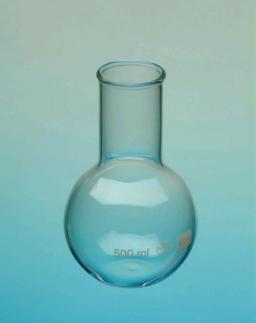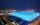# Chemical parison

The blown parison (with shape of a sphere) have a volume 1.5 liters. What is its surface?

S =  633.69 cm2

### Step-by-step explanation:Did you find an error or inaccuracy? Feel free to write us. Thank you!Tips to related online calculators
Do you know the volume and unit volume, and want to convert volume units?

#### You need to know the following knowledge to solve this word math problem:

We encourage you to watch this tutorial video on this math problem:

## Related math problems and questions:

• Cube surface and volumeThe surface of the cube is 500 cm2, how much cm3 will be its volume?
• Cube 6Volume of the cube is 216 cm3, calculate its surface area.
• Sphere A2VThe surface of the sphere is 241 mm2. What is its volume?
• SphereThe surface of the sphere is 12100 cm2, and the weight is 136 kg. What is its density?
• Cube surfce2volumeCalculate the volume of the cube if its surface is 150 cm2.
• EightEight small Christmas balls with a radius of 1 cm have the same volume as one large Christmas ball. What has a bigger surface: eight small balls, or one big ball?
• Cube zoomHow many percents do we increase the volume and surface of the cube if we magnify its edge by 38 %?
• Area to volumeIf the surface area of a cube is 486, find its volume.
• Magnified cubeIf the lengths of the cube's edges are extended by 5 cm, its volume will increase by 485 cm3. Determine the surface of both the original and the magnified cube.
• Cube-shaped boxThe cube-shaped box is filled to the brim with 2 liters of milk. Calculate the edge and surface of the box.
• Area of a cubeCalculate the surface area of a cube if its volume is equal to 729 cubic meters.
• Sphere areaA cube with an edge 1 m long is a circumscribed sphere (vertices of the cube lie on a sphere's surface). Find the surface area of the sphere.
• Iron sphereIron sphere has weight 100 kg and density ρ = 7600 kg/m3. Calculate the volume, surface, and diameter of the sphere.
• The cubeThe cube has a surface area of 216 dm2. Calculate: a) the content of one wall, b) edge length, c) cube volume.
• Cube 6The surface area of one wall cube is 1600 cm square. How many liters of water can fit into the cube?
• Triangular prismThe regular triangular prism has a base edge of 8.6 dm and a height of 1.5 m. Finf its volume and surface area.
• The volumeThe volume of the sphere is 1 m3, what is its surface?